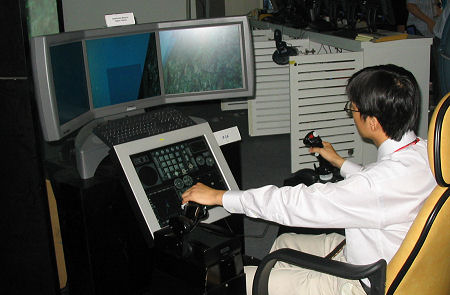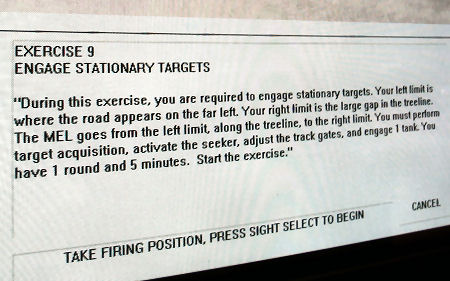Search IntMath
Close

# Learning by simulations

By Murray Bourne, 24 Feb 2006

The airline industry were amongst the first to use simulations for learning.

At Asian Aerospace today, I asked 3 of the simulator exhibitors to give me a fly, but they politely refused. Damn - it would have been a lot of fun:One of the exhibitors was showing this scenario-based simulation for military training:Learning should be more like this in educational institutions. Instead, it is often boring lectures followed by more boring lectures. Let's move away from this 19th century model now that we are in the 21st.

Be the first to comment below.

### Comment Preview

HTML: You can use simple tags like <b>, <a href="...">, etc.

To enter math, you can can either:

1. Use simple calculator-like input in the following format (surround your math in backticks, or qq on tablet or phone):
a^2 = sqrt(b^2 + c^2)
(See more on ASCIIMath syntax); or
2. Use simple LaTeX in the following format. Surround your math with $$ and $$.
$$\int g dx = \sqrt{\frac{a}{b}}$$
(This is standard simple LaTeX.)

NOTE: You can mix both types of math entry in your comment.